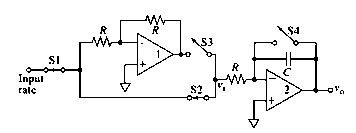# (a) Interpretation: The function of the operational amplifier 1 should be determined. Concept introduction: The operational amplifier is a high gain device and it amplifies the voltage. Generally, a voltage amplifier has a single output and differential input. So, basically, an operational amplifier produces an output much higher than the input voltage. The output voltage could be thousands of times higher than the input voltage. Here, the given electronic circuit is as below:### Principles of Instrumental Analysis

7th Edition
Douglas A. Skoog + 2 others
Publisher: Cengage Learning
ISBN: 9781305577213### Principles of Instrumental Analysis

7th Edition
Douglas A. Skoog + 2 others
Publisher: Cengage Learning
ISBN: 9781305577213

#### Solutions

Chapter 3, Problem 3.25QAP
Interpretation Introduction

## (a)Interpretation:The function of the operational amplifier 1 should be determined.Concept introduction:The operational amplifier is a high gain device and it amplifies the voltage. Generally, a voltage amplifier has a single output and differential input. So, basically, an operational amplifier produces an output much higher than the input voltage. The output voltage could be thousands of times higher than the input voltage.Here, the given electronic circuit is as below:Interpretation Introduction

### (b)Interpretation:The function of the operational amplifier 2 should be determined.Concept introduction:The operational amplifier is a high gain device and it amplifies the voltage. Generally, a voltage amplifier has a single output and differential input. So, basically, an operational amplifier produces an output much higher than the input voltage. The output voltage could be thousands of times higher than the input voltage.Here the given electronic circuit is as belowInterpretation Introduction

### (c)Interpretation:The output voltage during the interval Δt1 should be described and plotted. Where Δt1 is the first period when switches S1 and S2 are closed and switch S4 opens. The function of the operational amplifier 2.Concept introduction:The operational amplifier is a high gain device and it amplifies the voltage. Generally, a voltage amplifier has a single output and differential input. So, basically, an operational amplifier produces an output much higher than the input voltage. The output voltage could be thousands of times higher than the input voltage.Here the given electronic circuit is as belowInterpretation Introduction

### (d)Interpretation:The output voltage during the second interval Δt should be described and plotted. Where Δt is the second period when switches S2 opens and S3 closes.Concept introduction:The operational amplifier is a high gain device and it amplifies the voltage. Generally, a voltage amplifier has a single output and differential input. So, basically, an operational amplifier produces an output much higher than the input voltage. The output voltage could be thousands of times higher than the input voltage.Here the given electronic circuit is as belowInterpretation Introduction

### (e)Interpretation:The output voltage Vo at the end of the measurement cycle is given by vo=k× input rate should be shown.Concept introduction:The operational amplifier is a high gain device and it amplifies the voltage. Generally, a voltage amplifier has a single output and differential input. So, basically, an operational amplifier produces an output much higher than the input voltage. The output voltage could be thousands of times higher than the input voltage.Here the given electronic circuit is as belowInterpretation Introduction

### (f)Interpretation:The advantages and disadvantages of the given circuit over the normal amplifier should be described.Concept introduction:The operational amplifier is a high gain device and it amplifies the voltage. Generally, a voltage amplifier has a single output and differential input. So, basically, an operational amplifier produces an output much higher than the input voltage. The output voltage could be thousands of times higher than the input voltage.Here the given electronic circuit is as belowInterpretation Introduction

### (g)Interpretation:The scenario if the input signal gets changes with the slope during the measurement cycle should be determined.Concept introduction:The operational amplifier is a high gain device and it amplifies the voltage. Generally, a voltage amplifier has a single output and differential input. So, basically, an operational amplifier produces an output much higher than the input voltage. The output voltage could be thousands of times higher than the input voltage.Here the given electronic circuit is as belowInterpretation Introduction

### (h)Interpretation:The result obtained if the two-time intervals are separated by a time delay Δt3 should be determined.Concept introduction:The operational amplifier is a high gain device and it amplifies the voltage. Generally, a voltage amplifier has a single output and differential input. So, basically, an operational amplifier produces an output much higher than the input voltage. The output voltage could be thousands of times higher than the input voltage.Here the given electronic circuit is as belowInterpretation Introduction

### (i)Interpretation:The result if the two-time intervals were of different duration should be determined.Concept introduction:The operational amplifier is a high gain device and it amplifies the voltage. Generally, a voltage amplifier has a single output and differential input. So, basically, an operational amplifier produces an output much higher than the input voltage. The output voltage could be thousands of times higher than the input voltage.Here the given electronic circuit is as belowInterpretation Introduction

### Want to see the full answer?

Check out a sample textbook solution.

### Want to see this answer and more?

Experts are waiting 24/7 to provide step-by-step solutions in as fast as 30 minutes!*

*Response times may vary by subject and question complexity. Median response time is 34 minutes for paid subscribers and may be longer for promotional offers.